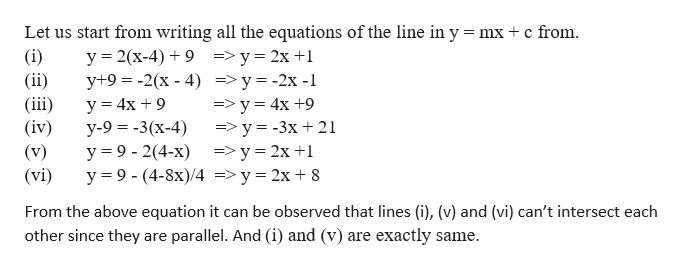There are six lines below. 3 of which intersect at the same point. which are they?1. y=2(x-4)+92. y+9=-2(x-4)3. y=4x+94. y-9=-3(x-4)5. y=9-2(4-x)6. y=9- (4-8x)/4

Question

There are six lines below. 3 of which intersect at the same point. which are they?

1. y=2(x-4)+9

2. y+9=-2(x-4)

3. y=4x+9

4. y-9=-3(x-4)

5. y=9-2(4-x)

6. y=9- (4-8x)/4

Step 1

We are given 6 lines and 3 of them intersect at the same ...help_outlineImage TranscriptioncloseLet us start from writing all the equations of the line in y mx c from (i) (ii) (iii (iv) (v) (vi) y 2(x-4)9=y 2x 1 y+9 -2(x - 4) =>y=-2x -1 у 3 4x + 9 y-9-3(x-4) у %39- 2(4-х) ->у%3D2х+1 y 9-(4-8x)/4 => y 2x 8 =>y 4x +9 =>y= -3x 21 From the above equation it can be observed that lines (i), (v) and (vi) can't intersect each other since they parallel. And (i) and (v) are exactly same. are fullscreen

Want to see the full answer?

See Solution

Want to see this answer and more?

Our solutions are written by experts, many with advanced degrees, and available 24/7

See Solution
Tagged in

Algebra